#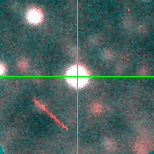V-102367 (J013357.98+304122.2)

## Target Notes### Classification: foreground

Unresolved from two LGGS stars: J013358.00+304122.3 of comparable brightness and J013358.02+304121.9 three magnitudes fainter in V and comparable brightness in BRI.

## Plots of Brightness and Color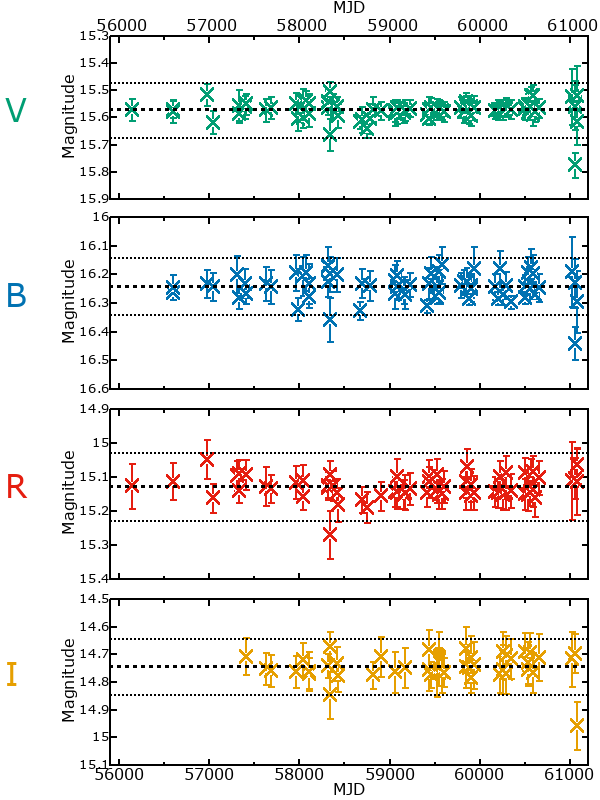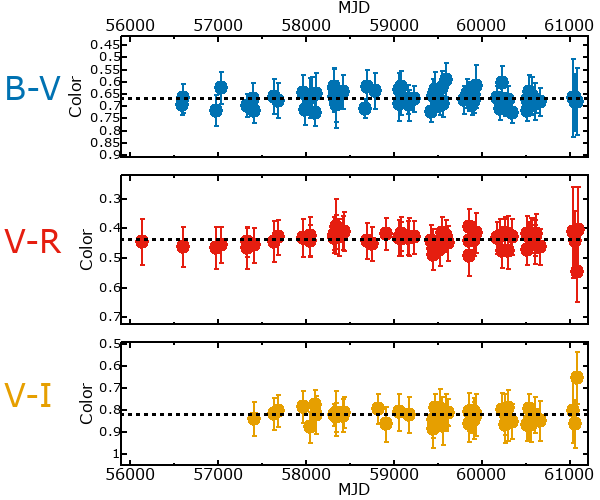## Variability

From the data available in this survey, it is not possible to tell with certainty if this star is variable.

### Correlation factors between bands

If the brightness variation is true variability then there should be a correlation between brightness changes recorded in different filter bands. The following table are the R-squared linear correlation factors calculated for the pairs of bands. The number in parentheses next to the R-squared value is the number of elements used to calculate the correlation factor. The number in square brackets next to the band is the range of magnitudes measured.

V [0.16 mag]B [0.11 mag]R [0.20 mag]
B [0.16 mag]0.45 (32)
R [0.20 mag]0.70 (29)0.32 (22)
I [0.12 mag]0.43 (16)0.39 (12)0.32 (13)

### Magnitude-Magnitude Plots

The plots below are provided to check the values in the table above and also to show any non-linear correlations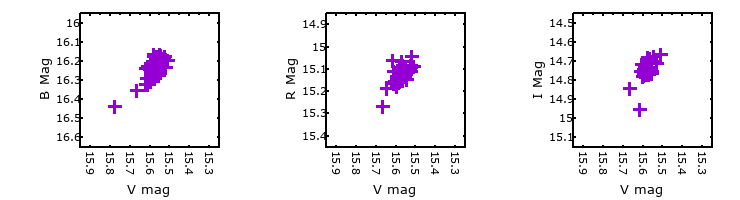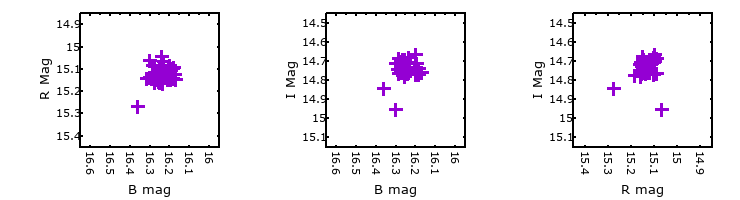### I & R Statistics

Welch & Stetson (1993, AJ, 105, 1813) present a method for varible star detection in CCD images from automated surveys. Data from two bands are compared and two statistics are calculated: R and I.

The I-statistic has an expectation value of zero for stars that are NOT variable. A larger non-zero I-value indicates a higher likelihood that the star is variable. The R statistic indicates how the color of the star changes as the variable grows brighter with R = 1.0 being no change in color.

R and I values have only been calculated if there are more than four (4) matched measurements of brightness in each band.
V
(I|R)
B
(I|R)
R
(I|R)
B
(I|R)
0.31 | 1.34
R
(I|R)
0.32 | 1.212.38 | 0.02
I
(I|R)
0.23 | 1.140.12 | 1.740.16 | 1.20